# 16-qubit IBM universal quantum computer can be fully entangled

## Abstract

Entanglement is an important evidence that a quantum device can potentially solve problems intractable for classical computers. In this paper, we prepare connected graph states involving 8 to 16 qubits on ibmqx5, a 16-qubit superconducting quantum processor accessible via IBM cloud, using low-depth circuits. We demonstrate that the prepared state is fully entangled, i.e., the state is inseparable with respect to any fixed partition.

## Introduction

Quantum computation has been an active research topic since the middle 90s with the invention of the Shor’s algorithm and many other important discoveries such as quantum error correction.1 For the last two decades, physical implementations of quantum computation have achieved significant progress. The fidelity of single and two-qubit gates exceeds 99%, reaching the threshold of fault-tolerant quantum computing.2 The number of qubits in both superconducting and trapped ions quantum computers are both greater than 20 now.3,4 It is projected that the number of qubits will approach to 50 or more in the next few years. At that time, the quantum computer may become more powerful than the fastest classical computer for some specific tasks, into the regime of the so-called quantum supremacy.5

The IBM Q is a quantum cloud service released by IBM. Its present backend devices include two processors with 5 superconducting qubits (ibmqx2 and ibmqx4), one 16-qubit processor (ibmqx5) and one 20-qubit processor (QS1_1).4 IBM recently announced that they have successfully built and tested a 20-qubit and a 50-qubit machine.4 The quantum cloud service of IBM provides high fidelity quantum gate operations and measurements. Hence, after the launch of the IBM Q, many groups tested it and performed quantum computing experiments on the cloud (for instance, see refs. 6,7,8,9,10,11,12).

Entanglement is considered to be the most nonclassical manifestation of quantum physics.13 It is also a critical resource for quantum information processing. Highly entangled states such as Bell states, GHZ (Greenberger–Horne–Zeilinger) states and cluster states14 have been applied in quantum teleportation, super-dense coding, one-way quantum computing15 and various quantum algorithms. The ability to produce highly entangled states is, therefore, one important step to demonstrate quantumness for quantum processors like ibmqx5. This task is, however, highly non-trivial due to the error accumulation of faulty gates.

In this paper, we wish to assess the quantumness and performance of the 16-qubit ibmqx5 device via the production of highly entangled states, namely the graph states, which is an important class of many-body entangled states that are widely used in one-way quantum computing, quantum error correction.15,16 We generate graph states that correspond to rings involving 8 to 16 qubits via IBM Q cloud service (ibmqx5), using optimized low-depth circuits that are tailored to the universal get set on ibmqx5. We detect full entanglement up to 16 qubits, using an entanglement criterion based on reduced density matrices. Qubits are fully entangled in the sense that the state involves all physical qubits and is inseparable with respect to any fixed partition.

## Results

### Graph states and entanglement

Graph state17 is a generalization of cluster state introduced in 2001,14 which is the resource state of one-way quantum computing15 and quantum error correction.16 GHZ state is an example of graph state and has been demonstrated in superconducting qubit system.18 However, GHZ state is fragile. Some other graph states are very robust to local operations, such as local measurements and noises. In order to disentangle the cluster state of N qubits, N/2 local measurements are needed.14 Because of this nice feature, we decide to generate and detect linear cluster states in the IBM cloud service ibmqx5.

X, Y, Z denote the Pauli operators. An undirected graph G(V, E) includes a set of vertices V and a set of edges E. A graph state that correspond to an undirected graph G(V, E) is a $$\left| V \right|$$-qubit state that has the form

$$\left| G \right\rangle = \mathop {\prod}\limits_{\left( {a,b} \right) \in E} {\kern 1pt} U_{ab}\left| + \right\rangle ^{ \otimes V},$$
(1)

where Uab is a control-Z operator acting on qubits a and b,19 and

$$\left| \pm \right\rangle = \frac{1}{{\sqrt 2 }}\left( {\left| 0 \right\rangle \pm \left| 1 \right\rangle } \right)$$
(2)

are eigenvectors of the X operator.

An equivalent definition, the graph state that corresponds to G(V, E), is the unique common eigenvector (of eigenvalue 1) of the set of independent commuting operators:

$$K_a = X^aZ^{N_a} = X^a\mathop {\prod}\limits_{b \in N_a} {\kern 1pt} Z^b,$$
(3)

where the eigenvalues to Ka are +1 for all aV, and Na denotes the set of neighbor vertices of a in G.19 As implied by the first definition, a n-qubit graph state can be prepared by the following steps.

1. 1.

Initialize the state to $$\left| + \right\rangle ^{ \otimes n}$$ by applying n Hadamard gates to $$\left| 0 \right\rangle ^{ \otimes n}$$;

2. 2.

For every (a, b) E, apply a control-Z gate on qubits a and b; the order can be arbitrary.

Entanglement of general mixed states was discussed by Werner in 1989.20 Since then, many entanglement criteria were proposed; among them the widely used ones include the partial transpose criterion13,21,22 and the symmetric extension criterion.23

A bipartite state ρAB on the Hilbert space $${\cal H} = {\cal H}_A \otimes {\cal H}_B$$ is said to be separable if ρAB can be written as

$$\rho _{AB} = \mathop {\sum}\limits_i {\kern 1pt} p_i\rho _A^i \otimes \rho _B^i,$$
(4)

where $$\rho _A^i$$ and $$\rho _B^i$$ are quantum states of the system A and B, respectively, with pi ≥ 0 and $$\mathop {\sum}\nolimits_i {\kern 1pt} p_i = 1$$. Otherwise ρAB is entangled. For a state ρ of a many-body system, for any fixed bipartition AB of the system, if ρ is entangled with respect to the partition AB, then the entanglement of the many-body state ρ can also be examined via its subsystems. That is, if the subsystems are all entangled, the whole system must be also entangled.

To be more concrete, consider a 4-qubit subsystem ρA,B,C,D in an n-qubit system. Suppose that we perform two local operations OA and OD on qubit A and D respectively, and then obtain the reduced density matrix of qubit B and C by tracing out qubit A and D. The reduced density matrix for qubits B and C reads

$$\rho _{B,C}^\prime = tr_{A,D}\left( {\frac{{O_AO_D\rho _{A,B,C,D}O_D^\dagger O_A^\dagger }}{{tr\left( {O_AO_D\rho _{A,B,C,D}O_D^\dagger O_A^\dagger } \right)}}} \right).$$
(5)

The entanglement of $$\rho _{B,C}^\prime$$ can be determined by using entanglement monotones such as negativity and concurrence, which, in the 2-qubit case, has non-zero values if and only if the system is entangled.13,22 If $$\rho _{B,C}^\prime$$ is entangled, we can conclude that in the original system, there could not exist a separation with qubit B and C on different sides. In other words, if the original system is biseparable with respect to a fixed partition, the qubit B and C must be on the same side. Otherwise, we will be able to create entanglement between the two separable parties with only local operations, which is not possible.13

For an n-qubit system {q1, q2, …, qn}, if we can show that among the n-qubit pairs (q1, q2), …, (qn−1, qn), (qn, q1), n − 1 of them must be on the same side in a separation, then we may conclude that there is no possible separation, and that the system is a n-qubit entangled state (meaning that the state is not biseparable with respect to (w.r.t.) a fixed partition, and that it involves all qubits). The (minimal) number of circuit configurations needed in this approach is 34(n − 1), which grows linear with respect to n. This method is far more efficient compared to a full n-qubit tomography, which requires exponential number of configurations.

### Graph states on ibmqx5

ibmqx5 is a 16-qubit superconducting quantum processor. It allows independent single-qubit operations with fidelity >99% and control operations with fidelity 95–97% (see Fig. 1) marked as the edges in the connectivity map (see Fig. 2). That is, controlled NOT (CNOT) operations with qubit a as the control qubit and b as the target is allowed if and only if a → b is an edge in the map.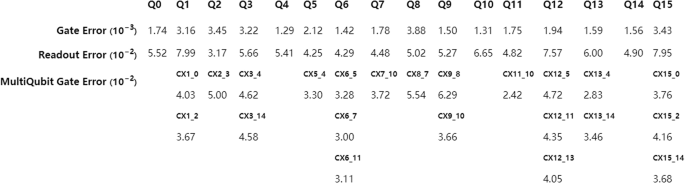Fig. 1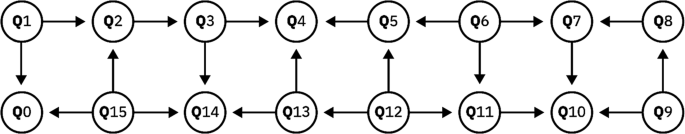Fig. 2

In our experiment, as shown in Fig. 3, the following five graph states are employed. The first state is a 8-qubit graph state involving qubits q5–q12 that corresponds to a ring of length 8; the second one is a 10-qubit state involving qubits q4–q13 corresponding to a ring of length 10; the third one involves qubits q3–q14 and corresponds to a ring of length 12; the fourth one involves qubits q2–q15 and corresponds to a ring of length 14; the fifth one involves all the 16 qubits. We employ these particular graph states based on the following considerations. First, these states are genuinely entangled and will remain entangled after tracing out a large number of qubits. Second, research has shown that one-dimensional (1D) cluster states are robust against decoherence, meaning that it would be more likely to find entanglement in a rather large graph state close to a 1D chain, compared to GHZ states and two-dimensional (2D) graph states.24 At last, even rings are two-edge colorable; as a result, on the 16-qubit ibmqx5, these “even-ring” states could be prepared using low-depth circuits (see Fig. 4).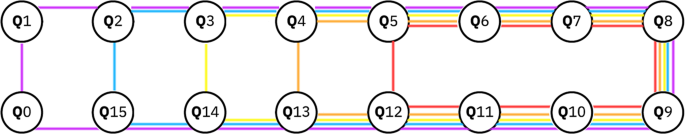Fig. 3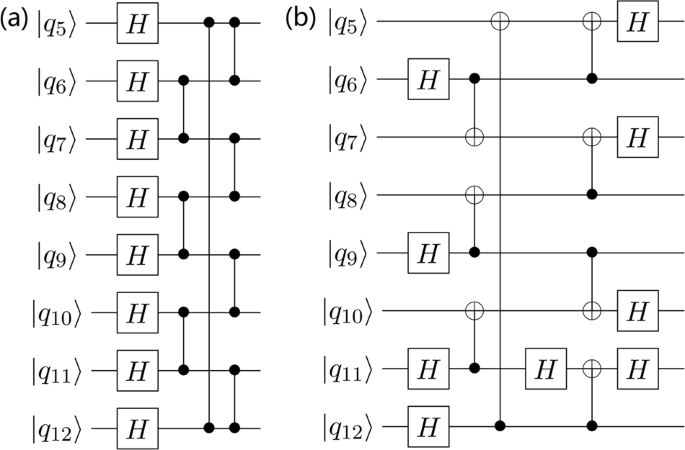Fig. 4

To prepare the desired graph state, we start from the circuit implied by the definition of graph states (see Fig. 4a). The control-Z gates are implemented using a CNOT gate and two Hadamard gates. We then optimize this circuit by adjusting the order of commuting gates and removing redundant Hadamard gates (see Fig. 4b). The circuit that we implemented are shown in Fig. 4b and Fig. 5a–d.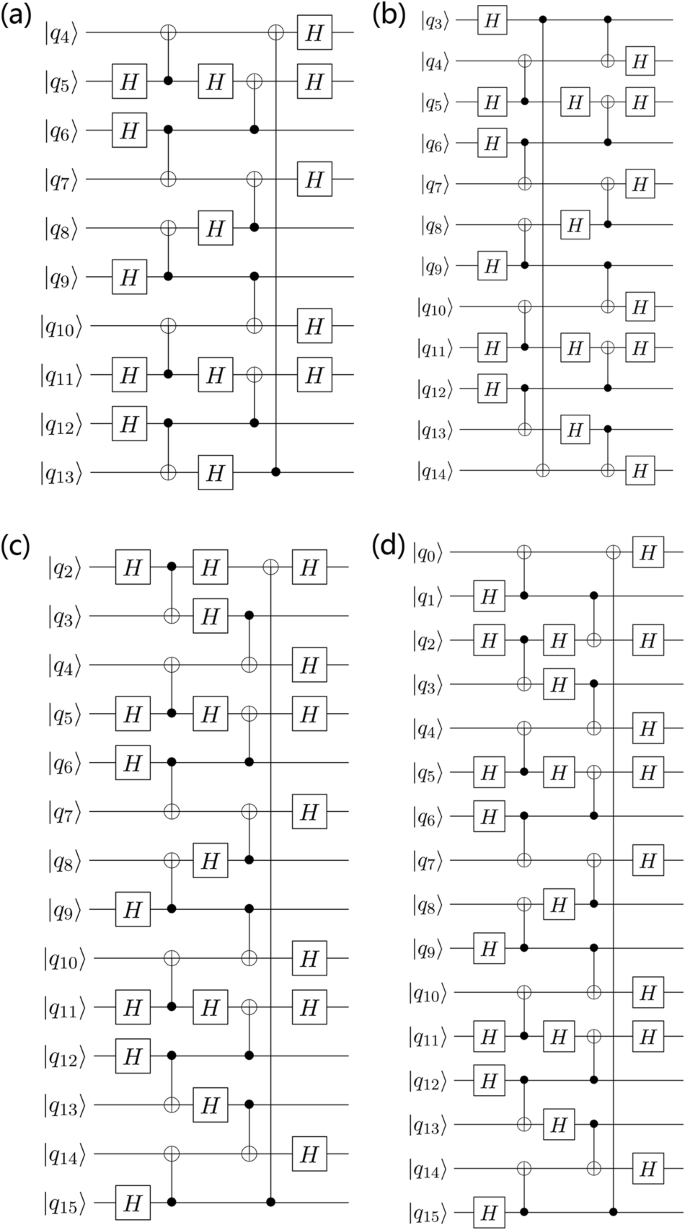Fig. 5

### Experimental results

For each n-qubit ring state, n partial tomographies are performed for every subsystem with 4 qubits that forms a chain in the ring. For example, for the 8-qubit graph state, the 8 subsystems are (q5, q6, q7, q8), (q6, q7, q8, q9), …, (q12, q5, q6, q7). For every state, 34n experimental configurations are used; 2048 measurements are taken under each configuration. The n 4-qubit reduced density matrices are obtained using the maximum likelihood method proposed by Smolin et al.25

Due to Eq. (3), for a ring graph state, each 4-qubit density matrix of neighboring four qubits, as illustrated in Fig. 6, is given by

$$\rho _{A,B,C,D} = \frac{1}{4}\left( {I + Z_AX_BZ_C} \right)\left( {I + Z_BX_CZ_D} \right).$$
(6)Fig. 6

Then, for each 4-qubit density matrix, we apply the local operations $$O_A = {\textstyle{{Z_A + I} \over 2}}$$ and $$O_D = {\textstyle{{Z_D + I} \over 2}}$$ and calculate the negativity of the resulting 2-qubit subsystem. For instance, we may choose (q5, q6, q7, q8) as our subsystem; after applying OA and OD to q5 and q8 respectively, we will trace out q5 and q8, and measure the negativity of the remaining subsystem, (q6, q7). We choose $$O_A = {\textstyle{{Z_A + I} \over 2}}$$ and $$O_D = {\textstyle{{Z_D + I} \over v}}$$ for the following reason. If ρ is graph state, and the 4-qubit subsystem corresponds to 4 vertices that form a chain in the graph, then the resulting 2-qubit state is a maximally entangled state

$$\left| \phi \right\rangle = \frac{1}{{\sqrt 2 }}\left( {\left| 0 \right\rangle \left| + \right\rangle + \left| 1 \right\rangle \left| - \right\rangle } \right).$$
(7)

Therefore, for a state close to this graph state, we should expect the resulting 2-qubit state to have a negativity significantly greater than 0. The results are plotted in Fig. 7.Fig. 7

For the 8-qubit graph state, the measured negativities are all significantly greater than 0. For the 10-qubit graph state, 9 out of 10 measured negativities are significantly greater than 0. Based on our argument above, both the 8-qubit state and 10-qubit state are fully entangled.

In the 12-qubit case, as shown in Fig. 7c, 10 out of 12 measured negativites are significantly non-zero. The two zeros come from (q9, q10) and (q14, q3) pairs. Therefore, there is only one possible separation, namely {q10, q11, q12, q13, q14} | {q3, q4, q5, q6, q7, q8, q9}. Should this be true, the reduced density matrix of qubits q8,q9,q10,q11 should also be separable with the separation {q8, q9} | {q10, q11}. In that case, its partial transpose with respect to qubit q8 and q9 must be positive. However, with respect to this partial transpose, ρq8,q9,q10,q11 has negativity 0.0391 ± 0.0039 (standard deviation estimated via bootstrapping). Therefore, this possibility is ruled out with very high confidence. We can now conclude that the 12-qubit graph state is fully entangled.

In the 14-qubit case, as shown in Fig. 7d, 12 out of 14 measured negativites are significantly greater than 0. Here, we may apply the same trick again. The only possible separation is {q2, q3, q4, q5, q6, q7, q8, q9, q12, q13, q14} | {q10, q11}. In this case, subsystem {q8, q9, q10, q11} should have zero negativity with respect to the partial transpose on q8 and q9. However, the measured negativity is 0.0698 ± 0.0048 (standard deviation estimated via bootstrapping). Hence, this possibility is ruled out with very high confidence. We may conclude that this state is fully entangled.

In the 16-qubit case, as shown in Fig. 7e, 15 out of 16 measured negativites are significantly greater than 0. As argued above, this means that this state is not biseparable w.r.t. a fixed partition, thereby showing that all 16 qubits in ibmqx5 are in full entanglement.

It may be noted that the subsystem of qubits {q8, q9, q10, q11} yields close-to-zero negativity in 3 out of 4 experiments. This can be due to relatively high readout errors or gate errors involving these qubits, which is compatible with the measured parameters provided by IBM website4 (see Fig. 1). For instance, the CNOT gate between q10 and q11 has the largest error among all possible CNOT gates, while the readout errors of q10 and q11 are also above the average level (6.5%).

### Further exploration of the 16-qubit state

The results above could be understood as an ability to generate localized entanglement on physically neighboring qubits.26 That is, neighboring qubits can be put into entanglement by performing ideal local operations on the 16-qubit state. Using the same data obtained above, we will show that localized entanglement on qubits with distance 2 and 3 could also be generated.

Suppose {E, A, B, C, D, F} is a 6-qubit subsystem that forms a chain. We first apply $$O_E = {\textstyle{{Z_E \pm I} \over 2}}$$ and $$O_F = {\textstyle{{Z_F \pm I} \over 2}}$$ on E and F respectively (four possibilities). On our data, this can effectively be done by first postselecting 0s on qubits E and F before calculating the tomography of {A, B, C, D}. Next, $$O_B = {\textstyle{{X_B + I} \over 2}}$$ and $$O_D = {\textstyle{{Z_D + I} \over 2}}$$ are performed (see Fig. 8). At last, B, E, F and D are traced out, while the negativity in subsystem {A, C} is calculated. If the 16-qubit state is perfect, this resulting system would be maximum entangled.Fig. 8

Based on data obtained in previous experiments, we have calculated the corresponding negativity for each 6-qubit subsystem and shown them in Table 1. Using this method, we have identified localized entanglement in 13 out of 16 pairs of qubits with distance 2.

To generate localized entanglement on qubits with distance 3, we may apply the same OE and OF, and then apply $$O_B^\prime = {\textstyle{{X_B + I} \over 2}}$$ and $$O_C^\prime = {\textstyle{{X_C + I} \over 2}}$$ (see Fig. 9). The negativity of subsystem {A, D} would be calculated. Again, if the 16-qubit state is perfect, these two qubits would be maximum entangled; therefore, we should expect a non-zero negativity if the actual state is close to the theoretical one.Fig. 9

Among 16 pairs of qubits with distance 3, we have identified localized entanglement in 6 pairs of them. The results based on our data is presented in Table 2.

## Discussion

We have prepared graph states of 8, 10, 12, 14 and 16 qubits on the 16-qubit ibmqx5 processor and demonstrated that these graph states are not biseparable w.r.t. any fixed partition. In particular, we have realized full entanglement using all 16 qubits. Moreover, we have demonstrated the ability to create localized entanglement on qubit pairs with distance 3 and 4 from this 16-qubit entangled state. In our approach of detecting nonseparability, we only have to measure the reduced density matrix of up to 4 qubits, and the size of reduced density matrix does not scale with the total qubit number, i.e., our method is efficient and scalable. In our experiments, graph states do not have high fidelity because of the large number of qubits, e.g., the fidelity of the 12-qubit graph state is lower than 0.44. (This upperbound is obtained by computing the fidelity between each 4-qubit subsystem and the theoretical result and taking the minimum.) However, the negativity of 4-qubit reduced density matrix decays gently with respect to the qubit number, which implies that the error per qubit weakly depends on the qubit number. It is a strong evidence that ibmqx5 is capable of generating highly entangled states and demonstrates the computer’s quantumness. In computational tasks such as one-way quantum computing, graph state with decaying fidelity is acceptable, and the computing is fault tolerant as long as the error per qubit is lower than a threshold.27,28

## Data availability

The experimental data that support the findings of this study29 are available in figshare with the identifier https://doi.org/10.6084/m9.figshare.6790781.

## References

1. 1.

Nielsen, M. A. & Chuang, I. Quantum Computation and Quantum Information (Cambridge University Press, Cambridge, 2002).

2. 2.

Barends, R. et al. Superconducting quantum circuits at the surface code threshold for fault tolerance. Nature 508, 500–503 (2014).

3. 3.

Friis, N. et al. Observation of entangled states of a fully controlled 20-qubit system. Phys. Rev. X 8, 021012 (2018).

4. 4.

IBM Q experience. https://quantumexperience.ng.bluemix.net/qx/devices. Accessed 27 December 2017.

5. 5.

Preskill, J. Quantum computing and the entanglement frontier. arXiv preprint arXiv:1203.5813 (2012).

6. 6.

Alsina, D. & Latorre, J. I. Experimental test of mermin inequalities on a five-qubit quantum computer. Phys. Rev. A 94, 012314 (2016).

7. 7.

Devitt, S. J. Performing quantum computing experiments in the cloud. Phys. Rev. A 94, 032329 (2016).

8. 8.

Berta, M., Wehner, S. & Wilde, M. M. Entropic uncertainty and measurement reversibility. New J. Phys. 18, 073004 (2016).

9. 9.

Rundle, R. P., Mills, P. W., Tilma, T., Samson, J. H. & Everitt, M. J. Simple procedure for phase-space measurement and entanglement validation. Phys. Rev. A 96, 022117 (2017).

10. 10.

Huffman, E. & Mizel, A. Violation of noninvasive macrorealism by a superconducting qubit: Implementation of a leggett-garg test that addresses the clumsiness loophole. Phys. Rev. A 95, 032131 (2017).

11. 11.

Hebenstreit, M., Alsina, D., Latorre, J. I. & Kraus, B. Compressed quantum computation using a remote five-qubit quantum computer. Phys. Rev. A 95, 052339 (2017).

12. 12.

Ferrari, D. & Amoretti, M. Demonstration of envariance and parity learning on the IBM 16 qubit processor. ArXiv e-prints (2018). 1801.02363.

13. 13.

Horodecki, R., Horodecki, P., Horodecki, M. & Horodecki, K. Quantum entanglement. Rev. Mod. Phys. 81, 865 (2009).

14. 14.

Briegel, H. J. & Raussendorf, R. Persistent entanglement in arrays of interacting particles. Phys. Rev. Lett. 86, 910 (2001).

15. 15.

Raussendorf, R. & Briegel, H. J. A one-way quantum computer. Phys. Rev. Lett. 86, 5188 (2001).

16. 16.

Raussendorf, R. & Harrington, J. Fault-tolerant quantum computation with high threshold in two dimensions. Phys. Rev. Lett. 98, 190504 (2007).

17. 17.

Hein, M., Eisert, J. & Briegel, H. J. Multiparty entanglement in graph states. Phys. Rev. A 69, 062311 (2004).

18. 18.

Song, C. et al. 10-qubit entanglement and parallel logic operations with a superconducting circuit. Phys. Rev. Lett. 119, 180511 (2017).

19. 19.

Hein, M. et al. Entanglement in graph states and its applications (2006). quant-ph/0602096.

20. 20.

Werner, R. F. Quantum states with einstein-podolsky-rosen correlations admitting a hidden-variable model. Phys. Rev. A 40, 4277 (1989).

21. 21.

Peres, A. Separability criterion for density matrices. Phys. Rev. Lett. 77, 1413 (1996).

22. 22.

Horodecki, M., Horodecki, P. & Horodecki, R. Separability of mixed states: necessary and sufficient conditions. Phys. Lett. A 223, 1–8 (1996).

23. 23.

Doherty, A. C., Parrilo, P. A. & Spedalieri, F. M. Distinguishing separable and entangled states. Phys. Rev. Lett. 88, 187904 (2002).

24. 24.

Hein, M., Dür, W. & Briegel, H.-J. Entanglement properties of multipartite entangled states under the influence of decoherence. Phys. Rev. A 71, 032350 (2005).

25. 25.

Smolin, J. A., Gambetta, J. M. & Smith, G. Efficient method for computing the maximum-likelihood quantum state from measurements with additive gaussian noise. Phys. Rev. Lett. 108, 070502 (2012).

26. 26.

Popp, M., Verstraete, F., Martín-Delgado, M. A. & Cirac, J. I. Localizable entanglement. Phys. Rev. A 71, 042306 (2005).

27. 27.

Nielsen, M. A. & Dawson, C. M. Fault-tolerant quantum computation with cluster states. Phys. Rev. A 71, 042323 (2005).

28. 28.

Raussendorf, R., Harrington, J. & Goyal, K. A fault-tolerant one-way quantum computer. Ann. Phys. 321, 2242–2270 (2006).

29. 29.

Wang, Y., Li, Y., Yin, Z. & Zeng, B. 16-qubit ibm quantum processor entanglement (2018). https://figshare.com/articles/16-qubit_IBM_quantum_processor_entanglement/6790781/1.

30. 30.

The IBM Q experience ibmqx5 backend. https://github.com/QISKit/ibmqx-backend-information/tree/master/backends/ibmqx5. Accessed 27 December 2017.

Download references

## Acknowledgements

We gratefully acknowledge the IBM Q team for providing us access to their 16-qubit platform. The views expressed are those of the authors and do not reflect the official policy or position of IBM or the IBM Quantum Experience team. Y.L. is supported by NSAF (Grant No. U1730449). Z.-Q.Y. is supported by the National Natural Science Foundation of China Grants 61771278, 11574176 and 11474177. B.Z. is supported by NSERC and CIFAR.

## Author information

Y.L., Z.-Q.Y. and B.Z. designed and conceived the study. Y.W. designed quantum circuits and performed the experiments. All authors wrote the manuscript.

Correspondence to Zhang-qi Yin.

## Ethics declarations

### Competing interests

The authors declare no competing interests.

## Additional information

Publisher's note: Springer Nature remains neutral with regard to jurisdictional claims in published maps and institutional affiliations.

## Rights and permissions

Open Access This article is licensed under a Creative Commons Attribution 4.0 International License, which permits use, sharing, adaptation, distribution and reproduction in any medium or format, as long as you give appropriate credit to the original author(s) and the source, provide a link to the Creative Commons license, and indicate if changes were made. The images or other third party material in this article are included in the article’s Creative Commons license, unless indicated otherwise in a credit line to the material. If material is not included in the article’s Creative Commons license and your intended use is not permitted by statutory regulation or exceeds the permitted use, you will need to obtain permission directly from the copyright holder. To view a copy of this license, visit http://creativecommons.org/licenses/by/4.0/.

Reprints and Permissions

## Further reading

• ### Experimental observation of classical analogy of topological entanglement entropy

• Tian Chen
• , Shihao Zhang
• , Yi Zhang
• , Yulong Liu
• , Su-Peng Kou
• , Houjun Sun
•  & Xiangdong Zhang

Nature Communications (2019)

• ### Genuine 12-Qubit Entanglement on a Superconducting Quantum Processor

• Ming Gong
• , Ming-Cheng Chen
• , Yarui Zheng
• , Shiyu Wang
• , Chen Zha
• , Hui Deng
• , Zhiguang Yan
• , Hao Rong
• , Yulin Wu
• , Shaowei Li
• , Fusheng Chen
• , Youwei Zhao
• , Futian Liang
• , Jin Lin
• , Yu Xu
• , Cheng Guo
• , Lihua Sun
• , Anthony D. Castellano
• , Haohua Wang
• , Chengzhi Peng
• , Chao-Yang Lu
• , Xiaobo Zhu
•  & Jian-Wei Pan

Physical Review Letters (2019)

• ### Bayesian deep learning on a quantum computer

• Zhikuan Zhao
• , Alejandro Pozas-Kerstjens
• , Patrick Rebentrost
•  & Peter Wittek

Quantum Machine Intelligence (2019)

• ### Toward large-scale fault-tolerant universal photonic quantum computing

• S. Takeda
•  & A. Furusawa

APL Photonics (2019)

• ### Resource-efficient verification of quantum computing using Serfling’s bound

• Yuki Takeuchi
• , Atul Mantri
• , Tomoyuki Morimae
• , Akihiro Mizutani
•  & Joseph F. Fitzsimons

npj Quantum Information (2019)